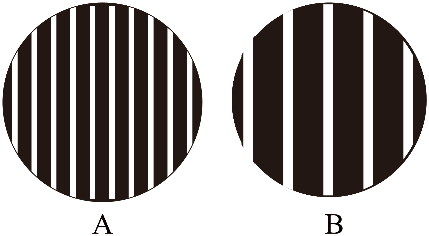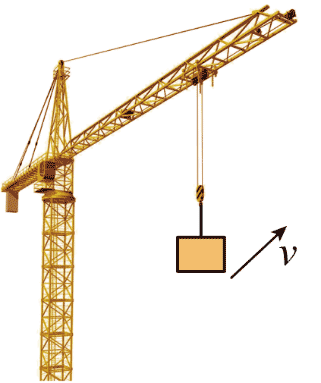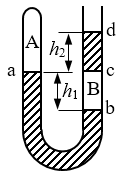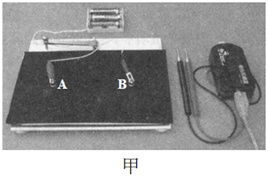## 宝山区2022学年高考模拟

•  2023/4/14
•  119 次
•  907 K

1．揭示原子核还可以再分的事实是

（A）电子的发现                                   （B）质子的发现

（C）天然放射性现象的发现              （D）中子的发现

【答案】

C

【解析】

A．电子的发现揭示了原子可以再分，A 错误；

B．质子的发现说明原子核中有质子，B 错误；

C．贝克勒尔发现了天然放射现象，说明了原子核内具有复杂的结构，揭示了原子核可以再分，C 正确；

D．中子的发现使人类对原子核的结构产生了更加深入的认识，D 错误。

2．如图 AB，分别为 a、b 两束单色光经过相同双缝干涉装置后在屏上形成的干涉图样，则

（A）a 光的波长大于 b 光的波长

（B）a 光的光子能量大于 b 光的光子能量

（C）在相同条件下，a 光比 b 光更容易发生明显的衍射现象

（D）若 a 光照射某金属能发生光电效应，则 b 光照射该金属也一定能发生光电效应

【答案】

B

【解析】

A．由图可知 a 光的干涉条纹间距小，而条纹间距与光的波长成正比，所以 a 光的波长小于 b 光的波长，A 错误；

B．根据波长与频率的关系可知，a 光的频率大于 b 光的频率，根据光子能量表达式 E = 可知 a 光的光子能量大于 b 光的光子能量，B 正确；

C．根据发生明显衍射的条件：狭缝或障碍物的尺寸与光的波长差不多或远小于光波的波长，可知波长越长越容易发生衍射，所以在相同条件下，b 光比 a 光更容易发生明显的衍射现象，C 错误；

D．因为 a 光的光子能量大于 b 光的光子能量，所以若 a 光照射某金属能发生光电效应，则 b 光照射该金属不一定能发生光电效应，D 错误。

3．如图所示，塔吊正在以速度 v 向右上方匀速提升重物，忽略空气阻力，则

（A）重物所受的合力指向右上方

（B）重物受到三个力的作用

（C）悬吊重物的钢丝绳一定竖直向上

（D）钢丝绳对重物的拉力逐渐变大

【答案】

C

【解析】

4．将氧气瓶由寒冷的室外搬到温暖的室内，并放置一段时间，瓶内氧气

（A）分子热运动的平均动能变小，压强变小

（B）分子热运动的平均动能变小，压强变大

（C）分子热运动的平均动能增大，压强变小

（D）分子热运动的平均动能增大，压强变大

【答案】

D

【解析】

5．如右图所示，直线 A、B 分别为电源 a、b 的路端电压与流过电源电流的关系图像。若将一定值电阻 R0 分别接到 a、b 两电源上，通过 R0 的电流分别为 IaIb，则

（A）Ia > Ib                             （B）Ia < Ib

（C）Ia = Ib                             （D）以上情况都有可能

【答案】

D

【解析】作出电阻的 UI 图线，电阻 R0UI 图线与电源的 UI 图线的交点表示电阻的工作状态，如图有三种可能，则知流过 R0 的电流 I 的大小关系不确定，无法比较大小。

6．如图所示，两条斜线分别为 a、b 两物体作直线运动的 v t 图像，已知 t = 0 时两物体在同一位置，则

（A）b 的速度始终比 a 的速度大

（B）b 的加速度始终比 a 的加速度大

（C）t = 5 s 时两物体相遇

（D）t = 10 s 时两物体相遇

【答案】

D

【解析】A．根据 v t 图像可知，t = 5 s 之前 b 物体的速度大于 a 物体，t = 5 s 之后 b 物体的速度小于 a 物体，A 错误；

B．v t 图像的斜率表示加速度，根据图像可知 b 的加速度始终比 a 的加速度小，B 错误；

C．t = 5 s 时两物体速度相等，v t 图线与时间轴围成的面积表示位移，根据图像可知，5 s 内 b 的位移大于 a 的位移，C 错误；

D．根据两物体的图像结合几何关系，可知 10 s 内两物体的位移相等，又因为两物体 0 时刻从同一位置出发，所以 t = 10 s 时两物体相遇，D 正确。

7．在用火箭把航天器送入太空发射的初始阶段，火箭通过燃烧消耗燃料向后吐着长长的“火舌”，推动着航天器竖直上升。设“火舌”产生的推动力大小保持不变，且不计空气阻力，则在这个过程中航天器的加速度大小

（A）一直增大              （B）一直减小

（C）一直不变              （D）如何变化，无法确定

【答案】

A

【解析】

8．探测器绕火星做匀速圆周运动，变轨后在周期较小的轨道上仍做匀速圆周运动，则

（A）探测器的线速度变小                  （B）探测器的角速度变小

（C）轨道半径变大                               （D）探测器受到的向心力变大

【答案】

D

【解析】

C．根据万有引力提供向心力，对探测器有 G= m$$\frac{{4{\pi ^2}}}{{{T^2}}}$$r，解得 T = 2π$$\sqrt {\frac{{{r^3}}}{{GM}}}$$。根据题意，探测器的周期变小，所以轨道半径变小，C 错误；

A．根据万有引力提供向心力，对探测器有 G= m$$\frac{{{v^2}}}{r}$$，解得 v = $$\sqrt {\frac{{GM}}{r}}$$。轨道半径变小，线速度变大，A 错误；

B．根据周期与角速度的关系 ω = $$\frac{{2\pi }}{T}$$ 可知，周期变小，角速度变大，B 错误；

D．根据万有引力定律，轨道半径变小，距离火星的距离变小，所以受到的引力变大，D 正确。

9．某 64 g 放射性元素经过 6 min，还有 2 g 尚未衰变，则该元素的半衰期为

（A）1.2 min         （B）1.5 min         （C）2 min             （D）3 min

【答案】

A

【解析】

10．如图所示，M 能在水平光滑滑杆上滑动，滑杆连架装在离心机上，用绳跨过光滑滑轮与另一质量为 m 的物体相连。当离心机以角速度 ω 在水平面内绕竖直轴转动时，M 离轴距离为 r，且恰能作匀速圆周运动。若 m 增至原来的 2 倍，保持 r 不变，为使 M 仍能作匀速圆周运动，则离心机的角速度要变为原来的

（A）1 倍              （B）$$\sqrt 2$$ 倍           （C）2 倍              （D）4 倍

【答案】

B

【解析】

m 增至原来的 2 倍，保持 r 不变，M 仍能作匀速圆周运动，同理有 2mg = Mω2r

11．如图所示，某列波在 t = 0 时刻的波形如图中实线，虚线为 t = 0.3 s（已知该波的周期 T > 0.3 s）时刻的波形图。已知 t = 0 时刻质点 P 正在做加速运动，则下列说法中正确的是

（A）波速为 10 m/s

（B）周期为 1.2 s

（C）t = 0.3 s 时刻质点 P 正向上运动

（D）在 0 ~ 0.1 s 内质点 P 运动的平均速度为 0.4 m/s

【答案】

A

【解析】

B．由题，t = 0 时刻质点 P 正在做加速运动，可知 P 的运动方向向上，则波沿 x 轴负方向传播。根据两时刻的波形，且 T > t，得到时间 t = $$\frac{3}{4}$$T，解得 T = 0.4 s。B 错误；

A．由图可知波长 λ = 4 m，则波速 v = $$\frac{\lambda }{T}$$ = 10 m/s。A 正确；

C．t = 0 时刻质点 P 的运动方向向上，在虚线为t = 0.3 s 时刻，质点 P 应处于负位移处且向下振动，C 错误；

D．在 0 ~ 0.1 s 内质点 P 运动向上振动，在 0 ~ 0.1 s 内即 $$\frac{T}{4}$$ 内位移大于一个振幅，即大于 4 cm，所以平均速度大于 0.4 cm/s。D 错误。

12．如图所示，一粗细均匀的 U 型玻璃管开口向上竖直放置，左、右两管都封有一定质量的理想气体 A、B，水银面 a、b 间的高度差为 h1，水银柱 cd 的长度为 h2，且 h2 = h1，a 面与 c 面恰处于同一高度。若在右管开口端取出少量水银，系统重新达到平衡，则

（A）A 气体的压强大于外界大气压强

（B）B 气体的压强变化量大于 A 气体的压强变化量

（C）水银面 c 上升的高度小于水银面 a 下降的高度

（D）水银面 a、b 间新的高度差小于右管上段新水银柱的长度

【答案】

B

【解析】

A．取出水银前，B 气体压强 pB = p0 + ρgh2，A 气体压强 pA = pBρgh1 = p0 + ρgh2ρgh1 = p0

C．由玻意耳定律可知 A、B 气体体积均变大。

D．设重新平衡后高度差变为 h1′、h2′，有 pB′ = p0 + ρgh2′，pA′ = pB′ – ρgh1′ = p0 + ρgh2′– ρgh1′，由于 pA′ < p0，所以 h1′ > h2′，即水银面 a、b 间新的高度差大于右管上段新水银柱的长度。D 错误。

B．由上面的分析：pA = pBρgh1pA′ = pB′ – ρgh1′，所以ΔpA = ΔpBρg(h1h1′)。而 A 体积增大，导致 h1 > h1′，所以 ΔpB > ΔpA。B 正确。

13．卢瑟福通过________实验，推断出原子的全部正电荷和几乎全部________都集中在原子中央一个很小的体积内，由此提出了原子的核式结构模型。

【答案】

α 粒子散射；质量

【解析】

14．如图，气缸中的气体膨胀时，推动活塞向外运动，若气体对活塞做的功是 4×104 J，气体的内能减少了 6×104 J，则在此过程中气体________（选填“吸收”或“放出”）的热量是________ J。

【答案】

【解析】

− 6×104 J = − 4×104 J + Q

Q = − 2×104 J

15．如图甲所示，一竖直放置的载流长直导线和 abcd 矩形导线框固定在同一竖直平面内，线框在长直导线右侧，且其长边与长直导线平行。在 t = 0 到 t = t1 时间内，长直导线中电流 I 随时间变化如图乙所示，图中箭头表示电流 i 的正方向，则线框中感应电流的方向为_____________；线框受到的安培力方向为_____________。

【答案】

【解析】

16．三根电阻相同的电阻丝连接成一个闭合的正三角形线框，O 为正三角形线框的中心。当强度为 I 的电流从 a 点流入 c 点流出时，ac 边在 O 点产生的磁场方向为__________（选填：“垂直于纸面向里”或“垂直于纸面向外”）。已知通电直导线在 O 点产生磁场的磁感应强度与导线中的电流强度成正比，若 ac 边在 O 点产生的磁场磁感应强度为 B，则整个线框在 O 点产生的磁场磁感应强度大小为_________。

【答案】

【解析】

（1）当电流从 a 点流入 c 点流出时，根据右手螺旋定则可知，ac 边在 O 点产生的磁场方向为垂直于纸面向里；

（2）三根电阻相同的电阻丝，ab 与 bc 串联，再与 ac 并联，所以 ab 与 bc中的电流为 ac 中的一半，又因为通电直导线在 O 点产生磁场的磁感应强度与导线中的电流强度成正比，根据右手螺旋定则和磁场矢量的叠加原理可知，ab 与 bc 中的电流在 O 点产生的磁垂直纸面向外，合磁感应强度与 ac 边在 O 点产生的磁感应强度相等，所以整个线框在 O 点产生的磁场磁感应强度大小为 0。

17．在电场中放置一光滑绝缘水平桌面，沿桌面上 x 轴方向电势分布如图中实线所示。一质量 m = 4×10−2 kg、电量 q = − 2×10−6 C的带负电小球，以 v0 = 2 m/s 的初速度在 x0 = − 1 m 处沿 x 轴正方向运动，则小球从开始运动到动能最大时电场力所做的功为________J；当小球速度为零时其位置坐标为________m。

【答案】

0.04；2 或 – 2

【解析】

（1）x 轴上从 – 3 m 到 0 电势升高，则电场方向沿 x 轴负方向，从 0 到 3 m 电势降低，则电场方向沿 x 轴正方向，带负电小球在 x0 = − 1 m 处沿 x 轴正方向运动，受到向右的电场力，电场力做正功，动能增加，从 0 点向右运动过程中电场力向左，电场力做负功，小球动能减小，所以小球在 0 点动能最大，则小球从开始运动到动能最大时电场力所做的功为 W = qU = q(φ−1φ0) = − 2×10−6×(2 − 4)×104 J = 0.04 J

（2）设小球速度为 0 时，其坐标为 x，从初位置到速度为零的过程，动能和电势能之和守恒，可得 $$\frac{1}{2}$$mv02 + −1 = ，解得 φ = − 2 V，由图像可知位置坐标为 2 m 或 – 2 m。

18．关于“用DIS描绘电场的等势线”实验

（1）如图甲所示，在一块平整的木板上，依次铺放白纸、________、导电纸。导电纸有导电物质的一面应该_________放置，用图钉将它们固定好。电极 A 与电源的正极相连作为“正电荷”，电极 B 与电源的负极相连作为“________”。本实验中使用的传感器是________传感器。

（2）如图乙所示，在电极 A、B 的连线上等距离地取 a、b、c、d、e 共 5 个基准点，若将传感器的两个探针分别接触图中的 b、p两点（c 为 AB 的中点，b、p 连线和 A、B 连线垂直），显示出 b、P 两点的电势差大于零，则传感器的“+”接线柱接在________点。此时若要采集到电势差为零，则应将接 p 的探针________（填“向左”或“向右”）移动。（3）如图乙所示，若传感器的两个探针分别接触 c 点和 d 点时的示数为 U1；分别接触 d 点和 e 点时的示数为 U2，则 |U1| ________ |U2|（选填“>”、“<”或“=”）。

【答案】

（1）复写纸；向上；负电荷；电压

（2）b；向左

（3）<

【解析】

（1）实验时探针是不能直接记录下等势点的位置，需要将复写纸铺在白纸上，以便在白纸上记录下等电势点；因为实验时，需将电极通过带导线的探针，以及导电纸形成一个闭合回路，所以导电纸导电的一面要向上；本实验是模拟等量异种电荷电场中的等势线，电极 A 与电源的正极相连作为“正电荷”，电极 B 与电源的负极相连作为“负电荷”；本实验需要找等势点，所以使用的传感器是电压传感器，因为等势点间的电势差为零。

（2）两个探针分别接触图中的 b、p 两点（c 为 AB 的中点，b、p 连线和 AB 连线垂直），显示出 b、P 两点的电势差大于零，因为电极 A 与电源的正极相连，根据等量异种电荷等势线的特点可知，b 点电势高于 p 点，因此传感器的“+”接线柱接在 b 点；将接 p 的探针向左移动，靠近电极 A 才能使电势差为零；

（3）根据等量异种电荷周围的电场特点可知，靠近两电极场强较大，根据 U = Ed，传感器的两个探针分别接触 c 点和 d 点时的示数为 U1；与接触 d 点和 e 点时的示数为 U2 的关系是 |U1| < |U2|。

19．在海滨游乐场有一种滑沙的娱乐活动。如图所示，人坐在滑板上从斜坡上离地高 24 m 的 A 点，由静止开始下滑，滑到斜坡底端 B 点后，沿水平滑道 BC 继续滑行。斜坡滑道与水平滑道间是平滑连接的，滑板与两滑道间的动摩擦因数均为 0.5，斜坡倾角 θ = 37°，不计空气阻力，重力加速度 g = 10 m/s2，sin37° = 0.6，cos37° = 0.8。

（1）求人在斜坡上下滑时的加速度大小；

（2）若人和滑板的总质量为 60 kg，求它们从 A 点滑到 B 点过程中损失的机械能；

（3）为了确保人身安全，水平滑道 BC 至少应该多长？

【答案】

（1）a1 =2 m/s2

（2）ΔE = 9600 J

（3）sBC = 16 m

【解析】

（1）由 mgsin37° − f1 = ma1f1 = μNN = mgcos37°

（2）解法一：

sAB = $$\frac{h}{{\sin 37^\circ }}$$ = $$\frac{{24}}{{0.6}}$$ m = 40 m

f1 = μmgcos37° = 0.5×60×10×0.8 N = 240 N

Wf1 = f1sAB = 240×40 J = 9600 J

vB2 = 2a1sAB，$$\frac{{1}}{{2}}$$mvB2 = $$\frac{{1}}{{2}}$$m·2a1sAB = 60×2×40 J = 4 800 J

ΔE = （14400 – 4800）J = 9600 J

（3）为了确保人身安全，人在水平滑道 BC 上要能静止下来：

f2 = ma2f2 = μmg，推得 a2 = μg = 0.5×10 m/s2 = 5 m/s2

vB2 = 2a1sABvB2 = 2a2sBC

20．如图所示，处于匀强磁场中水平放置的两根足够长、电阻不计的平行光滑金属导轨 MN 和 PQ 相距 L = 0.5 m。导体棒 ab、cd 与轨道垂直并保持良好接触，它们分别在大小相等、方向垂直导体棒的外力 F 作用下，沿着导轨各自朝相反方向，以速度 v0 匀速运动。导轨上接有阻值为 1 Ω 的电阻 R，在其两端接有电压表 V，此时电压表 V 的读数为 0.2 V。已知导体棒 ab、cd 的电阻 r 均为 0.5 Ω，它们的质量 m 均为 0.2 kg，匀强磁场磁感应强度的大小 B = 1 T、方向与导轨平面垂直。（1）问导体棒 ab 中的感应电流方向怎样？

（2）求导体棒 ab 两端的电压 U

（3）求外力 F 的功率 P

（4）问：若将作用在导体棒 ab、cd 上的外力 F 都撤去，则导体棒 ab 通过的最大位移 s 是多少？

【答案】

（1）由 a 指向 b

（2）Uab = 0.1 V

（3）P = 0.04 W

（4）s = 0.32 m

【解析】

（1）导体棒 ab 中的感应电流方向由 a 指向 b

（2）对于 R，有 I = $$\frac{U}{R}$$ = $$\frac{{0.2}}{1}$$ A = 0.2 A

I = $$\frac{{2{E_{{\rm{ab}}}}}}{{R + 2r}}$$，0.2 = $$\frac{{2{E_{{\rm{ab}}}}}}{{1 + 2 \times 0.5}}$$，Eab = 0.2 V

Uab = EabIr = （0.2 – 0.2×0.5）V = 0.1 V

（3）因为导体棒 ab 作匀速运动，所以有

F = F = BIL = 1×0.2×0.5 N = 0.1 N

Eab = BLv0，0.2 = 1×0.5×v0v0 = 0.4 m/s

（4）撤去 F 后，ab 棒仅受安培力 F 作用，

F = B·$$\frac{{2BLv}}{{R + 2r}}$$·L = $$\frac{{2{B^2}{L^2}v}}{{R + 2r}}$$

F = $$\frac{{2{B^2}{L^2}v}}{{R + 2r}}$$ = ma

$$\frac{{2{B^2}{L^2}\Delta {s_1}}}{{R + 2r}}$$ = m·Δv1，$$\frac{{2{B^2}{L^2}\Delta {s_2}}}{{R + 2r}}$$ = m·Δv2，$$\frac{{2{B^2}{L^2}\Delta {s_3}}}{{R + 2r}}$$ = m·Δv3，……

$$\frac{{2{B^2}{L^2}s}}{{R + 2r}}$$ = m(v0 – 0)，s = $$\frac{{m{v_0}(R + 2r)}}{{2{B^2}{L^2}}}$$，

s = $$\frac{{0.2 \times 0.4(1 + 2 \times 0.5)}}{{2 \times {1^2} \times {{0.5}^2}}}$$ = 0.32 m

2006 - 2023，推荐分辨率1024*768以上，推荐浏览器Chrome、Edge等现代浏览器，截止2021年12月5日的访问次数：1872万9823 站长邮箱